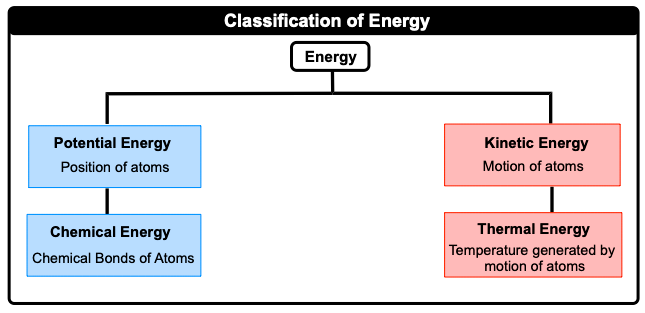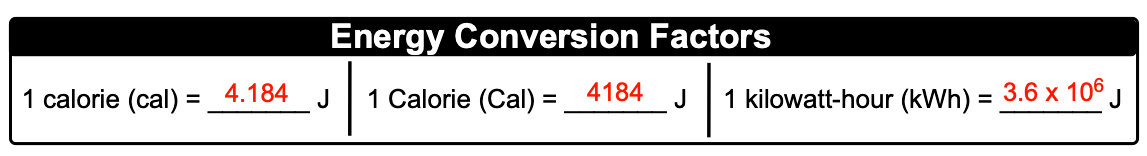Clutch Prep is now a part of Pearson
Ch.7 Energy, Rate and EquilibriumWorksheetSee all chapters

# Nature of Energy

See all sections
Sections
Nature of Energy
First Law of Thermodynamics
Endothermic & Exothermic Reactions
Bond Energy
Thermochemical Equations
Heat Capacity
Thermal Equilibrium (Simplified)
Hess's Law
Rate of Reaction
Energy Diagrams
Chemical Equilibrium
The Equilibrium Constant
Le Chatelier's Principle
Solubility Product Constant (Ksp)
Spontaneous Reaction
Entropy (Simplified)
Gibbs Free Energy (Simplified)

Thermochemistry is the study of matter and energy associated with chemical reactions or physical changes.

###### Classification of Energy

Concept #1: Nature of EnergyConcept #2: Energy Conversion FactorsExample #1: Which of the following statements deals with potential energy with nonchemical energy associated?

Practice: An energy efficient refrigerator uses 780 kWh of electrical energy per year. How many kilocalories of electricity does it use in three years?